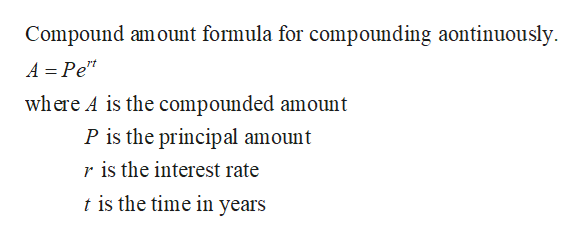# Section 2.3 (33)How long will it take a \$500 investment to be worth \$700  if it is continuously compounded at 10% per year? (Give the answer to two decimal places.)

Question
1 views

Section 2.3 (33)

How long will it take a \$500 investment to be worth \$700  if it is continuously compounded at 10% per year? (Give the answer to two decimal places.)

check_circle

Step 1

Recall the follow...help_outlineImage TranscriptioncloseCompound amount formula for compounding aontinuously. A = Pe* where A is the compounded amount P is the principal amount r is the interest rate t is the time in years fullscreen

### Want to see the full answer?

See Solution

#### Want to see this answer and more?

Solutions are written by subject experts who are available 24/7. Questions are typically answered within 1 hour.*

See Solution
*Response times may vary by subject and question.
Tagged in

### Math